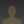# How to connect Voltage Sensor with Arduinoakanzler007 wrote 06/24/2020 at 11:37 • 3 min read • Like

One of the most important electrical measurements in most of these electronic devices is voltage. Measuring voltage is very important in projects/applications as giving wrong voltage to a device can lead to extreme malfunction of the device and the project. With this in mind Voltage Sensor module was created. It measures voltage and now there are different sensor modules which calculates voltages of different ranges.

A voltage sensor is a sensor is used to calculate and monitor the amount of voltage in an object. Voltage sensors can determine both the AC voltage and DC voltage level. The input of this sensor can be the voltage whereas the output is the switches, analog voltage signal, a current signal, an audible signal, etc. The pin diagram of the voltage Sensor Module is given below: -

The advantage of voltage sensors include:

• Small in weight and size.
• Personnel safety is high.
• Degree of accuracy is very high.
• It is non-saturable.
• Wide dynamic range.
• Eco-friendly.
• It is possible to combine both the voltage and current measurement into a single physical device with small and compact dimensions.

Working of the Voltage Sensor Module

Voltage Detection Sensor Module is a simple and very useful module that uses a potential divider to reduce any input voltage by a factor of 5. This allows us to use the Analog input pin of a microcontroller to monitor voltages higher than it capable of sensing. For example, with a 0V - 5V Analog input range, you are able to measure a voltage up to 25V. This module also includes convenient screw terminals for easy and secure connections of a wire. The Voltage Sensor is basically a Voltage Divider consisting of two resistors with resistances of 30KΩ and 7.5KΩ i.e. a 5 to 1 voltage divider.

The following image shows the schematic of the Voltage Sensor Module with an input voltage limit of 25V.

How to use Voltage Sensor Module

Now that we have seen a little about the Voltage Sensor, let us now proceed with interfacing a Voltage Sensor with Arduino and measure some external voltages.

The Arduino Voltage Sensor Interface is pretty straight forward.

• Connect the voltage to be measured to the screw terminal of the Voltage Sensor, connected the output of the voltage divider to the Arduino. That’s it.
• After interfacing the Voltage Sensor with Arduino, you can view the results on the serial monitor of the Arduino IDE.

Input and output can be calculated by using the following code: -

float Vout = 0.0;

float Vin = 0.0;

float R1 = 30000;

float R2 = 7500;

Vin = Vout * (R2/(R1+R2))

Vout = (analogvalue * 5 / 1024);

The application of voltages sensors include:

• Power failure detection.
• Safety switching.
• Temperature control.
• Power demand control.
• Fault detection.

Voltage sensors are included in many of the best Arduino starter kits, as they are very useful in many electronics projects. Get Additional Information on Voltage Sensor Module and keep your electronic projects safe.

This voltage sensor module is very useful in finding the voltage of a battery. If you want to know more specifications about the battery like what is AH, WH and other technicalities Click Here.

This blog has been written in reference to the blogs published by electronichubs.org and components101.com.

## Discussions